# CBSE Class 10 Science Periodic Classification Of Elements Assignment Set B

Read and download free pdf of CBSE Class 10 Science Periodic Classification Of Elements Assignment Set B. Get printable school Assignments for Class 10 Science. Standard 10 students should practise questions and answers given here for Chapter 5 Periodic Classification Of Elements Science in Grade 10 which will help them to strengthen their understanding of all important topics. Students should also download free pdf of Printable Worksheets for Class 10 Science prepared as per the latest books and syllabus issued by NCERT, CBSE, KVS and do problems daily to score better marks in tests and examinations

## Assignment for Class 10 Science Chapter 5 Periodic Classification Of Elements

Class 10 Science students should refer to the following printable assignment in Pdf for Chapter 5 Periodic Classification Of Elements in standard 10. This test paper with questions and answers for Grade 10 Science will be very useful for exams and help you to score good marks

### Chapter 5 Periodic Classification Of Elements Class 10 Science Assignment

Question :  Answer the questions on the basis of the following table, name the family of H, A, C. (1)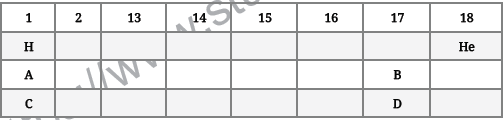a. Alkali
b. alkaline earth metal
c. Halogen
d. Noble

Question :  Nitrogen and phosphorus belong to the group 15 of the periodic table. Which of these will be more electronegative?
a. Nitrogen
b. Both are equally electronegative
c. None of them are electronegative
d. Phosphorus

Question : Which of the following statement is true?
Statement A: Electron affinity of fluorine is more than that of chlorine.
Statement B: Carbon monoxide is an acidic oxide.
a. Both the Statement A and B are true
b. Neither statement A nor statement B is true
c. Statement A is true, B is false
d. Statement B is true, A is false

Question : The law of octaves was proposed by
a. Mendeleev
b. Newland
c. Dobereiner
d. Lothar Meyer

Very Short Answer type Questions :

Question. Give reason why noble gases are placed in a separate group in the modern periodic table.
Answer : It is because they have their outermost shell completely filled and resemble with each other.

Question. Give the number of elements in 2nd and 5th period in the modern periodic table.
Answer : In 2nd period, there are 8 elements. In 5th period, there are 18 elements.

Question. Write the formula which is used to determine the maximum number of electrons that a shell can accommodate.
Answer : 2n2 , where n is the shell number.

Question. What is the valency of phosphorus with atomic number 15?
Answer : P(2, 8, 5) has 5 valence electrons, therefore its valency is equal to 3.

Question. Find the atomic number of the element whoseelectronic configuration is 2, 8, 5.
Answer : Atomic number = 2 + 8 + 5 = 15.

Question. The atomic number of three elements A, B and C are 12, 18 and 20 respectively. State giving reason, which two elements will show similar properties.
Answer : A(12): 2, 8, 2, B(18): 2, 8,8, C(20): 2, 8, 8, 2 A and C belong to the same group because they have same number of valence electrons.

Question. The formula of magnesium oxide is MgO. State the formula of barium nitrate and barium sulphate, if barium belongs to same group as magnesium.
Answer : The valency of Mg is 2. The valency of Ba is also 2 as they belong to the same group. Formula of Barium nitrate is Ba(NO3)2, and that of Barium sulphate is BaSO4.

Question. Out of the three elements P, Q and R having atomic numbers 11, 17 and 19 respectively, which two elements will show similar properties and why?
Answer : P(11): 2, 8, 1; Q(17): 2, 8, 7; R(19): 2, 8, 8, 1 P and R will show similar chemical properties because they have the same number of valence electrons.

Question. Why are H, Li, Na and K placed in group-1?
Answer : It is because they have one valence electron.

Question. State the modern periodic law of classification of elements.
Answer : Modern Periodic Law: It states ‘properties of elements are a periodic function of their atomic number’.

Question. What is the valency of an element with atomic number 35?
Answer : Atomic number is 35, it has electronic configuration of 2, 8, 18, 7. It has 7 valence electrons, therefore its valency is equal to 1.

Question. Out of Li and K, which will have stronger metallic character and why?
Answer : K will have more metallic character because it can lose electrons easily due to its bigger atomic size and less effective nuclear charge.

Question. Write the atomic numbers of two elements X and Y
having electronic configurations 2, 8, 2 and 2, 8, 6,
respectively.
Answer : X = 2 + 8 + 2 = 12
Y = 2 + 8 + 6 = 16

Question. Name any two elements which were discovered later after the formation of Mendeleev’s periodic table.

Question. What were the criteria used by Mendeleev in creating his periodic table?
Answer : Increasing order of atomic mass and same formula of oxides and hydrides.

Question. What would be the nature of oxides formed by the elements on the right side of periodic table?
Answer : On right hand side of the periodic table, most of them are non-metals, therefore they form acidic oxides.

Question. Write the number of horizontal rows in the modern periodic table. What are these rows called?
Answer : There are 7 horizontal rows in the modern periodic Table. These rows are called periods.

Question. The electronic configuration of two elements X and Y are 2, 8, 7 and 2, 8, 8, 3 respectively. Write the atomic numbers of X and Y.
Answer : X = 2 + 8 + 7 = 17
Y = 2 + 8 + 8 + 3 = 21

Question. Write one difference between Group 1 and Group 2 elements?
Answer : Group 1 elements has 1 valence electron whereas group 2 elements has 2 valence electrons.

Question. Predict the maximum number of valence electrons possible for atoms in the first period of the periodic table.
Answer : Maximum number of valence electrons in 1st period is 2.

Question. How many vertical columns are there in the modern periodic table and what are they called?
Answer : There are 18 vertical columns in Modern Periodic Table. They are called groups.

Question. An element has atomic number 17. To which group and period does it belong to?
Answer : X(17): 2,8,7 is the electronic configuration. It has 7 valence electrons. It belongs to 17th group and 3rd period.

Question. What is a metalloid? Name any two of them.
Answer : Metalloids are those elements which resembles both with metals as well as non-metals e.g., Boron and Silicon.

Answer : It is because all the elements discovered at that time could not be classified as Dobereneire’s triads.

Question. The atomic numbers of three elements X, Y and Z are 3, 11 and 17 respectively. State giving reason which two elements will show similar properties.
Y = 2,8, 1; Z = 2, 8, 7
X and Y will show similar properties due to same number of valence electrons.

Question. Write two reasons responsible for the late discovery of noble gases.
Answer : a. They are inert i.e., least reactive.
b. They are less abundant in nature except Argon.

Question. Define Newlands law of octaves.
Answer : Newlands Law of Octaves: When elements are arranged in increasing order of their atomic mass, every eighth element resembles with the first.

Question. An element A has atomic number 16. To which group and period does it belong to?
Answer : A(16) has electronic configuration: 2, 8, 6. It has 6 valence electrons and three shells. It belong to Group 16, 3rd period.

Question. Arrange the following metals in decreasing order of their atomic size:
Ca, Mg, Ba, Be
Answer : Ba > Ca > Mg > Be is the decreasing order of atomic size.

Question. State the modern periodic law for the classification of elements. How many (a) groups, (b) periods are there in Modern Periodic Table?
Answer : Properties of elements are a periodic function of their atomic numbers. There are 18 groups and 7 periods in modern periodic table.

Question. Elements in Periodic table show periodicity in properties. List any four such properties.
Answer : Valence electrons, Valency, Atomic size, Metallic character.

Question. Name any two pairs of elements which were adjusted by Newlands in the same slot.
Answer :  (i) Co and Ni,
(ii) Ce and La

Question. Why does atomic size decreases as we move from left to right along a period in a periodic table?
Answer : It is because one proton and one electron is being added successively, therefore effective nuclear charge increases, atomic size decreases.

Question. List any two properties of elements belonging to the first group of modem periodic table.
Answer : a. They have 1 valence electron.
b. Their valency is equal to 1.

Question. State Mendeleev’s periodic law.
Answer : Mendeleev’s Periodic Law: Properties of elements are a periodic function of their atomic mass.

Question. Which has larger atomic radius, K(19) or Ca(20)?

Question. What is the valency of silicon with atomic number 14?
Answer : Si(2, 8, 4), the number of valence electrons in Si is 4. Therefore, its valency is 4.

Question : How many periods and groups are present in the long form of periodic table?
Answer :  The vertical columns in the periodic table are known as groups while the horizontal rows are known as periods. The long form of periodic table has seven periods and
eighteen groups.

Question : Atomic number of an element is 16. Write its electronic configuration. Find the number of valence electrons and its valency.
Answer :  Since, atomic number of the given element is 16. Hence, the element is sulphur(S). It is kept in group VI A or group 16 in modern periodic table.Number of valence electrons in sulphur are 6, present in M-shell. Its valency is 2 as it requires 2 electrons to complete its octet or achieve the nearest noble gas configuration.

Question : Name two elements you would expect to show chemical reactions similar to magnesium. What is the basis for your choice?
Answer : Calcium and Strontium would show chemical reactions similar to magnesium. They have the same number of electrons in the outermost shell.It is the electrons that take part in a chemical reaction.

Question : Where would you locate the element with electronic configuration 2, 8 in the modern periodic table?
Answer :  The element with an electronic configuration of (2,8) have completely filled valence shell. It has two shells. Therefore, it belongs to second period and group 18 (noble or inert gas) in the modern periodic table. Group number = valence shell electron + 10 = 8 +10 =18.

Question : Carbon (atomic number 6) and silicon (atomic number 14) are elements in the same group of the periodic table. Give the electronic arrangements of the carbon and silicon atoms and state the groups in which these elements occur. (3)
Answer : The required information may be given in a tabular form as follows: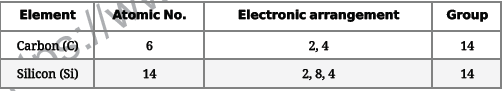Question : Write down the electronic configuration of elements with atomic numbers 2, 14, 17, 19. Indicate the group of the periodic table to which they belong.
Answer :  The information is being given in a tabular form.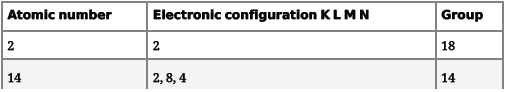Question : Compare and contrast the arrangement of elements in Mendeleev's Periodic table and the Modern Periodic table.
1) In both the elements are arranged in groups and periods.
2) In both similar elements are placed in same group.
3) Both the classification make the study of elements simple and systematic.
Differences.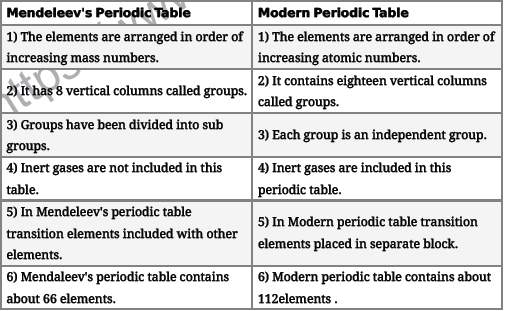Question : Given below are few elements of the modern periodic table. Atomic number of the element is given in the parenthesis
A (4) , B(9), C(14), D(19), E(20)
i. Select the elements that has one electron in the outermost shell. Also write the electronic configuration of this element.
ii. Which two elements amongst these, belong to the same group? Give reason for your answer.
iii. Which two elements amongst these belong to the same period? Which one of the two has bigger atomic radius?
Answer :  i. D (19) has one electron is its outermost shell. Electronic configuration of the element is given below. The element is potassium written as K, it belong to group I A in periodic table.ii. A(4) and E(20) belongs to same group as they have same number of valence electrons. A is beryllium symbol Be and E is calcium Symbol Ca. Both belong to group II A in the periodic table.iii. A(4) and B(9) belong to same period and A(4) has bigger radius than B(9). Also the elements D(19) and E(20) belong to same period and D(19) has bigger radius than E(20).

Question. The electrons in the atoms of four elements A, B, C and D are distributed in three shells havingl, 3, 5 and 7 electrons in the outermost shell respectively. State the period in which these elements can be placed in the modern periodic table. Write the electronic configuration of the atoms of A and D and the molecular formula of the compound formed when A and D combine.
Answer : A: 2, 8, 1
B: 2, 8, 3
C: 2, 8, 5
D: 2, 8, 7g
They belong to the 3rd period.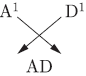Question. Examine elements of the third period: Na, Mg, Al, Si, P, S, Cl and Ar Answer the following:
a. Choose (i) Metals, (ii) Non-metals out of these elements.
b. On which side of periodic table we find (i) metals (ii) non-metals.
c. Name metalloids out of the elements given above.
Where are they located in the periodic table?
Answer : a. (i) Na, Mg, A1 are metals.
(ii) P, S, Cl, Ar are non-metals.
b. (i) Metals are placed on the left hand side and middle part of the periodic table.
(ii) Non-metals are placed on the right hand side of periodic table.
c. Silicon is a metalloid. They are located between metals and non-metals at the border line in a zigzag manner.

Question. Two elements P and Q belong to the same period of the modern periodic table and are in Group-1 and Group-2 respectively. Compare their following characteristics in tabular form:
a. The number of electrons in their atoms.
b. The size of their atoms.
c. Their metallic character.
d. Their tendency to lose electrons.
e. The formula of their oxides.
f. The formula of their chlorides.
Answer : a. P has 1 valence electron, Q has 2 valence electrons.
b. P is bigger than Q.
c. P is more metallic than Q.
d. P can lose electrons more easily than Q.
e. P2O and QO
f. PCl and QCl2

Question. Explain the variation in the following properties of the elements in periodic table:
a. Atomic radius in the periodic table,
b. Metallic character in a period,
c. Valency in a group.
Answer : a. Atomic radius and Metallic character increases down the group. Valency remains the same in a group.
b. Atomic size and metallic character decrease along
a period from left to right in a period.

Question. Write the main aim of classifying elements. Name the basic property of elements which is used in the development of Modern Periodic Table. State the Modern Periodic Law. On which side (part) of the Modern Periodic Table do you find metals, metalloids and non- metals?
Answer : Classification is done so as to study the properties of 118 elements easily. Modern periodic table is based on atomic number of the atom an element. ‘Properties of elements are a periodic function of their atomic numbers.’ Metals are placed on the left and middle, non-metals are placed on the right and Metalloids are placed on the border line between metals and nonmetals in a zig-zag manner.

Question. The position of three elements A, B and C in the Periodic Table is shown below:Giving reasons, explain the following:
a. Element ‘A’ is a non-metal.
b. Element ‘B’ has a larger atomic size than element ‘C’.
c. Element ‘C’ has a valency of 1.
Answer : a. A is a non-metal because it has 7 valence electrons, it can gain one electron to form anion.
b. B has less electrons and protons, less forces of attraction between the nucleus and valence electrons, therefore it is bigger in size.
c. C can gain 1 electron to become stable, therefore its valency is equal to 1.

Question. Atoms of seven elements A, B, C, D, E, F and G have a different number of electronic shells but have the same number of electrons in their outermost shells. The elements A and C combines with chlorine to form an acid and common salt respectively. The oxide of element A is liquid at room temperature and it is a neutral substance, while the oxides of the remaining six elements are basic in nature. Based on the above information, answer the following question:
a. What could the element A be?
b. Will elements A to G belong to the same period or same group of the periodic table?
c. Write the formula of the compound formed by the reaction of the element A with oxygen.
b. What would be the ratio of number of combining atoms in a compound formed by the combination of element A with carbon?
e. Which one of the given elements is likely to have the smallest atomic radius?
Answer : a. A is hydrogen.
b. A and G will belong to the same group.
c. H2O is the formula of the compound.
d. CA4 i.e., 1:4
e. A has smallest atomic radius.

Question. An element M with electronic configuration (2,8,2) combines separately with (NO3)-, (SO4)2- and (SO4)3-  radicals. Write the formula of the three compounds so formed. To which group and period of the Modern Periodic Table does the element M belong to? Will M form covalent or ionic compounds? Give reason to justify your answer.
Answer : M(2, 8, 2). It has valency equal to 2.M belongs to group 2.
It belongs to 3rd period.
M will form ionic compound because M can easily lose electrons.
Bond will be formed by transfer of electrons.

Question. Given below are some elements of the Modern Periodic Table. Atomic number of the element is given in the parentheses:
A(4), B(9), C(14), D(19), E(20)
a. Select the element that has only one electron in the outermost shell. Also write the electronic configuration of this element.
b. Which two elements amongst these belong to the same group? Give reason for your answer.
c. Which two elements amongst these belong to the same period? Which among the two has bigger atomic radius?
Answer : a. D(19): 2,8,8,1 has 1 valence electron.
b. A(4): 2, 2 and E(20): 2, 8, 8, 2 belong to same group because they have the same number of valence electrons.
c. A(2, 2), B(2, 7) belong to the same period. A has bigger atomic radius than B.

Question. a. Why did Mendeleev leave gaps in his periodic table?
b. State any three limitations of Mendeleev’s periodic table.
c. How do the electronic configuration of atoms change in a period with increase in atomic number.
Answer : a. He left gaps for the new elements which are to be discovered.
b. (i) Increasing order of atomic mass could not be maintained.
(ii) Position of hydrogen was not justified.
(iii) Isotopes could not be placed in different slots due to different atomic mass but same properties.
c. Number of valence electrons keep on increasing along a period from left to right in a period.

Question. a. Predict which of the following will form anions and which will form cations.
(i) Na, (ii) Al, (iii) Cl, (iv) O
b Name two elements that are inert.
Answer : a. Cl and O will form anions i.e., Cl- and O2- Na and A1 will form cations i.e., Na+ and Al3+.
b. He and Ne are inert elements.

Question. Two elements X and Y have atomic 12 and 17 respectively.
a. Write the electronic configuration of both these elements.
b. Which type of bond will they form?
Answer : a. X: 2, 8, 2; Y: 2, 8,7
b. They will form ionic bond.

Question. Out of the elements H(1), Be(4), Na(11) and Mg(12).
a. Write the pair of elements having similar chemical properties.
b. State the group number of each pair,
c. Name one another element belonging to each of these groups.
Answer : a. Be(4) and Mg(12) have similar chemical properties.
H( 1) and Na(ll) have similar chemical properties.
b. Be and Mg belong to group 2, H and Na belong to group 1.
c. K belongs to group 1 and Ca belongs to group 2.

Question. Calcium is an element with atomic number 20. Stating the reason, answer each of the following questions:
a. Is calcium a metal or a non-metal?
b. Will its atomic radius be larger or smaller than that of potassium with atomic number 19?
c. Write the formula of its oxide.
Answer : a. Calcium is a metal because it can lose electrons to form cations.
b. Its atomic radius will be smaller due to more number of protons and electrons, more forces of attraction.
c. CaO

Question. a. How does electropositivity of elements gets affected as we move (i) down the group, (ii) across the period?
b. Which atomic property increases both ways: as we move across the period or down the group?
Answer : a. (i) Electropositivity increases down the group.
(ii) Electropositivity decreases across the period from left to right.
b. Atomic number increases across the period as well as down the group.

Question. Study the following table in which positions of six elements A, B, C, D, E and F are shown as they are in the modern periodic table: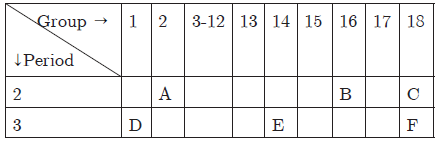On the basis of the above table, answer the following questions:
a. Name the element which forms only covalent compounds.
b. Which element is a metal with a valency of one?
c. Which element is a non-metal with a valency of two?
d. Out of D and E, which has a bigger atomic radius and why?
Answer : a. E forms only covalent compounds.
b. D is a metal with valency of 1.
c. B is a non-metal with valency of 2.
d. D has bigger atomic size because it has less effective nuclear charge.

Question. The electronic configuration of two elements A and B are 2, 8, 3 and 2, 8, 7 respectively. Find the atomic number of these elements. State the nature and formula of the compound formed by union of these elements.
Answer : A has 2 + 8 + 3 = 13 as its atomic number B has 2 + 8 + 7 = 17 as its atomic number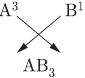AB3 is the formula of compound. It is an ionic compound.

Question. As we move across a period in the periodic table, what is gradation in the following properties: (a) Atomic size, (b) Atomic number, (c) Electronegativity?
Answer : a. Atomic size goes on decreasing across the period from left to right.
b. Atomic number goes on increasing along the period from left to right.
c. Electronegativity goes on increasing along a period from left to right.

Question. An element X belongs to 3rd period and group 16 of the Modern Periodic table.
a. Determine the number of valence electrons and the valency of X.
b. Molecular formula of the compound of X when it reacts with hydrogen and write its electron dot diagram.
c. Name the element X and state whether it is metallic or non-metallic.
Answer : a. X has 6 valence electrons and its valency is equal to 2.
b. H2X is its formula,
c. X is sulphur. It is a non-metallic element.

Question. Why do all elements of the
a. same group have similar properties?
b. same period have different properties?
Answer : a. It is due to same number of valence electrons which will decide the chemical properties.
b. They differ in number of valence electrons, therefore they differ in chemical properties. They have the same number of shells.

Important Revision Notes on Periodic Classification of Elements

¶ Elements : Substances containing atoms of only one type. eg. Na,Au,Mg etc.
– There are around 118 elements known to us.
¶ Elements are classified to make the study easy.
¶ Dobereiner’s Traids :When the elements were written in order of increasing atomic masses the atomic mass of the middle was the average of the atomic mass of the other two elements.
eg. Elements            AtomicMass
Ca                 40.1
Sr                 87.6
Ba                136.3
Limitations : Only three triads were recognised from the elements known at that time.
¶ Atomic mass of an element is the relative mass of its atom as compared widh the mass of a Carbon-12 atom taken as 12 units
¶ Newland’s law of octaves :
– Based on increasing atomic mass of elements.
– When elements are arranged it was found that every eighth element had properties similar to that of the first. eg properties of sodium and Lithium are the same.

Limitations :
– Applicable only upto Calcium
– Properties of new elements couldn’t fit in it.
– It some cases properties of the elements were not same as defined by octave.
– Worked well onlywith lighter elements.
Mendeleev's periodic law :– The properties of elements are the periodic function of their atomic mass.
Mendeleev's periodic table based on the chemical properties of elements.
Contain eight vertical columns called groups and seven horizontal rows called periods formMendeleev’s peridic table.
Achievements ofMendeleev’s Periodic table
– Elements with similar properties could be grouped together
– Some gaps were left for the undiscovered elements.
– Noble gases could be placed without disturbing the existing order.

Limitations:
– No fixed position for hydrogen
– No place for isotopes
– No regular trend in atomic mass.

Modern Periodic Table
Modern Periodic Law : Properties of elements are a periodic function of their atomic number.
– Atomic Number – denoted by Z and equals to the no. of protons in the nucleus of an atom.
– Modern periodic table contains 18 vertical columns known as groups and 7 horizontal rows known as periods.
– Elements in a group have valence electrons
– No. of the shells increases as we go down the group.
– Elements in a period have same number of shells.
– Each period marks a new electronic shell getting filled.
– No. of elements placed in a particular period depends upon the fact that how electrons are filled into various shells.
– Maximum no. of electrons that can be accomodated in a shell depend on the formula 2n2 where n is the no. of the given shell.
eg. k shell – 2 x 2 = 2 elements in the first period L shell – 2 x 2 = 8 elements in the second period.
– Position of the element in the periodic table tells about its reactivity.

Trends in the Modern Periodic Table
– Valency : No. of valence electrons present in the outermost shells.
– Atomic Size : Atomic size refers to radius of an atom.
– Atomic size or radius decreases in moving from left to right along a period due to increase in nuclear charge
– Atomic size increases down the group because new shells are being added as we go down the group.

Metallic Character : Metallic character means the tendency of an atom to lose electrons.
– Metallic character decreases across a period because the effective nuclear charge increases that means the tendency to lose electrons decreases.
– Metals are electropositive as they tend to lose electrons while forming bonds.
– Metallic character increases as we go down a group as the effective nuclear charge is decreasing. Non metals are electronegative. They tend to form bonds by gaining electrons.
– Metals are found on the left side of the period table while non-metals are towards the right hand side of the periodic table.
– In the middle we have semi-metals or metalloid because they exhibit some properties of both metals and non metals.
– Oxides of metals are basic in nature while oxides of non-metals are acidic in nature.
(Refer the table given on side page)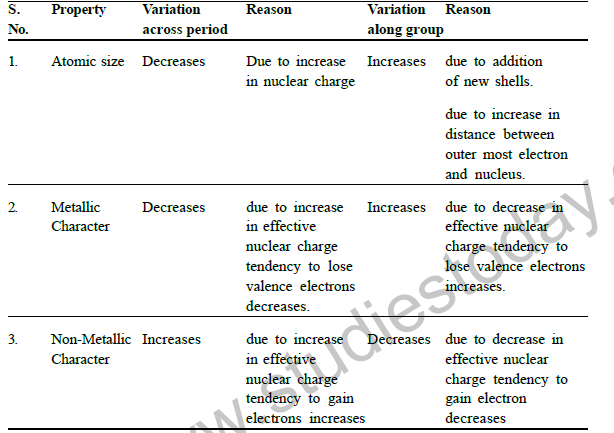Question 1: What is the position of hydrogen in the modern periodic table?

Question 2: Where are the isotopes of the same elements having different atomic masses placed in the periodic table ?

Question 3: An element M is the third group of the periodic table. Write the formula of its oxide?

Question 4: What is the valency of magnesium with atomic no. 12 and chlorine with atomic no. 17? `

Question 5: What is the difference in number of shell in magnesium and sulphur?

Question 6: On the basis of electronic configuration , how will you select

i)the terminating member in a period .

ii) the chemically similar elements .

Question 7: Give reason as to why the atomic radii of elements increase in a group while moving from top to bottom ?

Question 8: Element in a group of periodic table have similar chemical properties why ?

Question 9: Explain why atomic number is more important than atomic weight in determining chemical properties ?

Question 10: Where in periodic table do we find :

i) elements classified as non metal .

ii) elements forming negative ions .

iii) elements with high melting points .

iv) elements forming positive ions .

Question 11: In a group reactivity of metals increases while those of non metals decreases . Explain.

Question 12: Elements in a group of periodic table have similar chemical properties why

Question 13: Elements of group 18 are called zero group. Why?

Question 14: Write the electronic configuration of atoms of

A) potassium (K) B)argon (Ar) C)lithium (li) D)fluorine (F) E)chlorine (Cl)

Question 15: i) Why is potassium more reactive than lithium ?

ii) why is fluorine is more reactive than chlorine ?

iii) which is smaller in size Cl or Ar ?

iv) which is smaller in size Li or F ?

v) which is more electronegative F or Cl?

Question 16: The atomic no. of an element is 17.

i) what is its valency?

ii) Whether it is a metal or non-metal?

iii) Whether it is bigger or smaller in size then an element of atomic no.18?

iv) What type of bonds it will form with elements of group 18?

v) How would its oxide behave with litmus solution?

HOTS QUESTIONS

Question 1: an element has two electron in its M shell:

i) Identify the element. ?

ii) What type of ion will it form ?

iii) What will be the formula of its chloride ?

iv) Predict the solubility of its chloride ?

Question 2: which among the following elements whose atomic number are given below belong to the same period ? give the reason 17,10,20,12,19,15

Question 3: element X with atomic 12 and element Y with atomic number 17 reacts with hydrogen to form hydrides . Which of them is expected to have high melting points?

Question 4: why is position of hydrogen not justified in modern periodic table?

FORMATIVE ASSESSMENT IV

QUIZ

Question 1: Name the element with atomic number 12.

Question 2: Name a metal in making cans and a member of group14.

Question 3: Name the most electronegative element in the periodic table.

Question 4: Name the horizontal rows in the periodic table .

Question 5: On moving across the period , atomic size of the element increase or decrease.

Question 6: Who gave the classification on the basis of musical note .

Question 7: Name two elements belonging to group one which can be cut with the help of knife .

Question 8: What name is given to the elements belonging to group 2 of the periodic table and why?

Question 9: Name the lustrous non metal having 7 valence electron .

Question 10: Name the highly reactive metal that give violet colour to flame.

Question 11: Name the gas used in coloured advertising lights having 2 valence electron .

DEBATE AND DISCUSSION

A) Drawbacks of Mendeleev‘s and modern periodic table.

B) Achievements of Mendeleev‘s and modern periodic table.

C) Advantages of modern periodic table in understanding chemistry.

PROJECTS

1 Power point Presentation on the following topics:

1. Modern Periodic Table based on the similarity of properties of elements

2. Contribution by various Scientists towards the development of Periodic Table.

3. PERIODIC CLASSIFICATION

1.Making flash cards to study atomic numbers electronic configuration and other properties of elements.

2.Make an outline sketch of the Modern Periodic Table.

TOPIC 3: HOW DO ORGANISMS REPRODUCE? GIST OF THE LESSON

1) Reproduction: process by which living organism produce new individual of their own kind.

2) Creation of DNA copy: when the cell divides into two, each new cell gets a copy of each DNA or chromosomes.

3) Importance of variation: variations are created by DNA copying mechanism during sexual reproduction.

4) Asexual modes of reproduction:

a) Fission—binary & multiple fission

b) Fragmentation

c) Regeneration

d) Budding

e) Vegetative propagation

f) Spore formation

5) Sexual reproductiona)

In flowering plant

b) In human beings

6) Parts of flowers

7) Pollination: self and cross pollination

8) Fertilization: male and female germ cell fuses to form zygote.

9) Puberty: The age, when reproductive organs become functional,(in female 10-12 years, in male 13-14 years).

10) Male reproductive system in human beings.

 CBSE Class 10 Science Chemical Reactions and Equations Assignment
 CBSE Class 10 Science Acids Bases and Salts Assignment Set A CBSE Class 10 Science Acids Bases and Salts Assignment Set B CBSE Class 10 Science Acids Bases and Salts Assignment Set C
 CBSE Class 10 Science Metals and Non Metals Assignment Set A CBSE Class 10 Science Metals and Non Metals Assignment Set B
 CBSE Class 10 Science Carbon and its compounds Assignment Set A CBSE Class 10 Science Carbon and its compounds Assignment Set B CBSE Class 10 Science Carbon and its compounds Assignment Set C CBSE Class 10 Science Carbon and its compounds Assignment Set D CBSE Class 10 Science Carbon and its compounds Assignment Set E
 CBSE Class 10 Science Periodic Classification Of Elements Assignment Set A CBSE Class 10 Science Periodic Classification Of Elements Assignment Set B CBSE Class 10 Science Periodic Classification Of Elements Assignment Set C
 CBSE Class 10 Science Biology Life Process Respiration Assignment CBSE Class 10 Science Biology Nutrition Assignment CBSE Class 10 Science Biology Respiration Assignment CBSE Class 10 Science Biology Transport Enrichment Assignment CBSE Class 10 Science Biology Transportation Assignment CBSE Class 10 Science Life Processes Assignment Set A CBSE Class 10 Science Life Processes Assignment Set B CBSE Class 10 Science Life Processes Assignment Set C
 CBSE Class 10 Science Control and Coordination Assignment Set A CBSE Class 10 Science Control and Coordination Assignment Set B CBSE Class 10 Science Control and Coordination Assignment Set C CBSE Class 10 Science Control and Coordination Assignment Set D
 CBSE Class 10 Science How do Organisms Reproduce Assignment Set A CBSE Class 10 Science How do Organisms Reproduce Assignment Set B
 CBSE Class 10 Science Heredity and Evolution Assignment Set A CBSE Class 10 Science Heredity and Evolution Assignment Set B CBSE Class 10 Science Heredity and Evolution Assignment Set C
 CBSE Class 10 Science Light Reflection and Refraction Assignment Set A CBSE Class 10 Science Light Reflection and Refraction Assignment Set B CBSE Class 10 Science Light Reflection and Refraction Assignment Set C CBSE Class 10 Science Light Reflection and Refraction Assignment Set D CBSE Class 10 Science Light Reflection and Refraction Assignment Set E CBSE Class 10 Science Light Reflection and Refraction Assignment Set F
 CBSE Class 10 Science Human Eye and Colorful World Assignment Set A CBSE Class 10 Science Human Eye and Colorful World Assignment Set B CBSE Class 10 Science Human Eye and Colorful World Assignment Set C
 CBSE Class 10 Physics Electricity Assignment Set A CBSE Class 10 Physics Electricity Assignment Set B CBSE Class 10 Physics Electricity Assignment Set C CBSE Class 10 Science Electricity Assignment Set A CBSE Class 10 Science Electricity Assignment Set B CBSE Class 10 Science Electricity Assignment Set C
 CBSE Class 10 Science Magnetic Effects of Electric Current Assignment Set A CBSE Class 10 Science Magnetic Effects of Electric Current Assignment Set B CBSE Class 10 Science Magnetic Effects of Electric Current Assignment Set C CBSE Class 10 Science Magnetic Effects of Electric Current Assignment Set D
 CBSE Class 10 Science Sources of Energy Assignment Set A CBSE Class 10 Science Sources of Energy Assignment Set B CBSE Class 10 Science Sources of Energy Assignment Set C CBSE Class 10 Science Sources of Energy Assignment Set D
 CBSE Class 10 Science Our Environment Assignment Set A CBSE Class 10 Science Our Environment Assignment Set B CBSE Class 10 Science Our Environment Assignment Set C
 CBSE Class 10 Science Sustainable Management of Natural Resources Assignment Set A CBSE Class 10 Science Sustainable Management of Natural Resources Assignment Set B CBSE Class 10 Science Sustainable Management of Natural Resources Assignment Set C
 CBSE Class 10 Physics Revision Assignment Set A CBSE Class 10 Physics Revision Assignment Set B CBSE Class 10 Physics Revision Assignment Set C CBSE Class 10 Physics Revision Assignment Set D CBSE Class 10 Physics Revision Assignment Set E CBSE Class 10 Physics Revision Assignment Set F CBSE Class 10 Physics Revision Assignment Set G CBSE Class 10 Physics Revision Assignment Set H
 CBSE Class 10 Science Assignment CBSE Class 10 Science Assignments Collection CBSE Class 10 Science Energy Crossword Puzzle Assignment
 CBSE Class 10 Physics Question Bank CBSE Class 10 Physics Tungsten Assignment
 CBSE Class 10 Science Chemistry Cleaning Capacity of Soap Assignment CBSE Class 10 Science Chemistry Determination of pH Assignment CBSE Class 10 Science Chemistry Different Types of Chemical Reactions Assignment CBSE Class 10 Science Chemistry Displacement Reaction and Reactivity Series Assignment CBSE Class 10 Science Chemistry Properties of Acetic Acid Assignment CBSE Class 10 Science Chemistry Saponification Preparation of Soap Assignment CBSE Class 10 Science Chemistry Study of properties of HCI Acid and NaOH Assignment

Tags